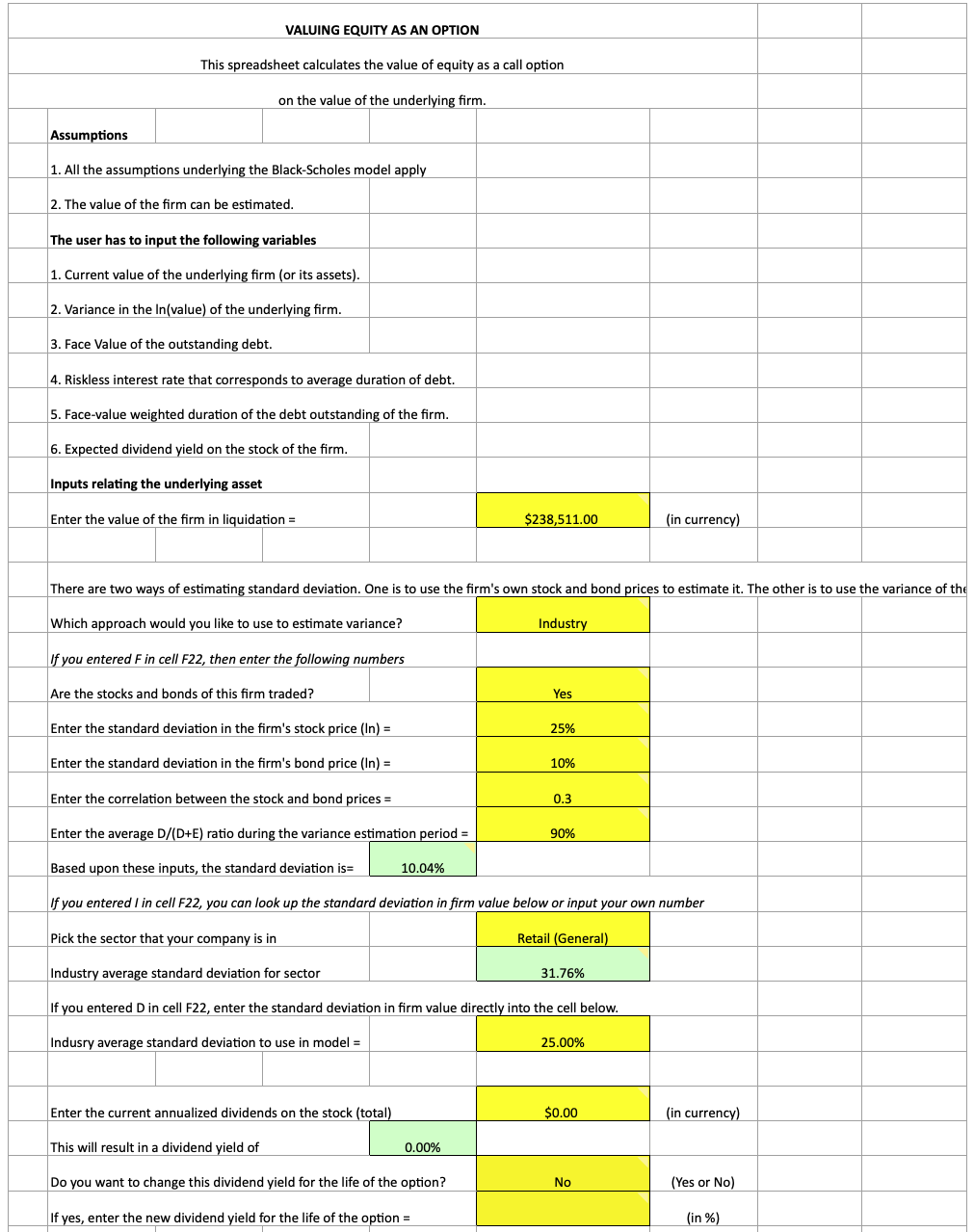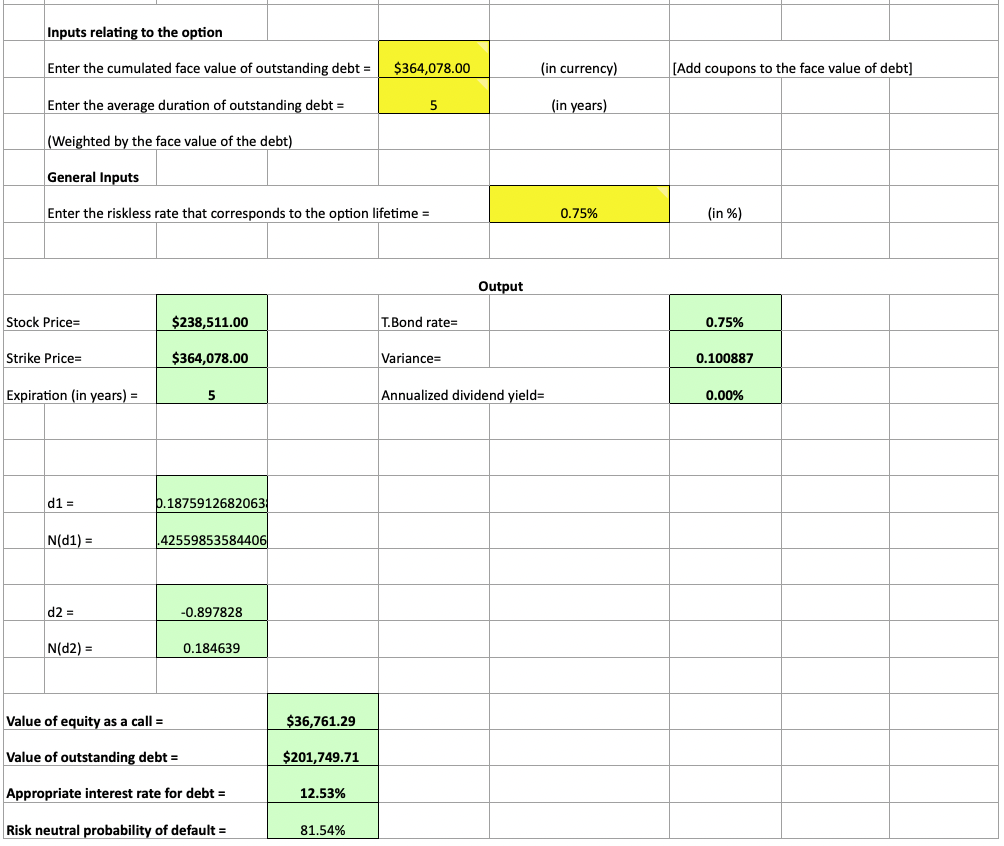# How to Value Distressed Firms through Black-Scholes

Using the Black-Scholes options pricing model, we can determine:

• the level of equity in a firm
• its value of outstanding debt
• the appropriate interest rate on the debt it has, and
• its risk neutral probability of default (i.e., bankruptcy)

This model would be most applicable to distressed, highly leveraged firms.

A distressed firm is one having trouble honoring its debt payback obligations. And naturally, a highly leveraged firm is one that has a high quantity of debt financing its assets.

The model discussed here uses the Black-Scholes framework to value the equity in a firm as a call option on the underlying value of the firm. It can be downloaded on NYU Stern Professor Aswath Damodaran’s website, located here, under the model name ‘equity.xls’.The assumptions of this model are based on that the value of the firm is already known from some type of alternative model (e.g., comparable company analysis (“comps“), precedent transactions, discounted cash flow), and that all the assumptions included in the Black-Scholes model hold true.

To value the equity in this particular firm, in addition to the appropriate interest rate on its debt, and risk neutral probability of default, we need the following inputs:

• The current value of the underlying firm or its assets
• The standard deviation of the value of the underlying firm for its particular industry
• Face value of the outstanding debt
• Current risk-free interest rate (i.e., long-term treasury bond yields)
• Duration of the outstanding debt
• Expected dividend yield of the firm’s stock

Within the spreadsheet you can select the industry of the company to find the appropriate values for different variables. A separate spreadsheet within the workbook contains data concerning the standard deviation of the equity in a firm and the standard deviation of firm value within each industry.

The data can also be entered manually, but having the industry data already available is helpful when easy access isn’t viable.

The model will use the values of a firm and apply them as their analogous option components.

The value of the firm is the stock price. The strike price is the firm’s debt level. The option’s expiration is the duration of the company’s outstanding debt. The standard deviation of the value of the firm is the variance of the stock’s volatility expressed, likewise, as a standard deviation.

The formulas follow the exact same definitions as those in the Black-Scholes model. In the end, we will have the following outputs:

• Value of equity
• Value of outstanding debt
• The appropriate interest rate for debt
• Risk neutral probability of default

## How Inputs Effect Outputs

### Current value of the underlying firm or its assets

A higher firm value will:

• Increase value of equity
• Increase value of outstanding debt
• Decrease the interest rate of debt
• Decrease the risk neutral probability of debt

### Standard deviation of the value of the underlying firm for its particular industry

A higher standard deviation of the value of the underlying firm for its relevant industry will:

• Increase value of equity
• Decrease value of outstanding debt
• Increase the interest rate of debt
• Increase the risk neutral probability of debt

### Face value of the outstanding debt

A higher amount of debt will:

• Decrease value of equity
• Increase value of outstanding debt
• Decrease the interest rate of debt
• Decrease the risk neutral probability of debt

### Current risk-free interest rate (i.e., long-term treasury bond yields)

A higher bond rate will:

• Increase value of equity
• Decrease value of outstanding debt
• Increase the interest rate of debt
• Decrease the risk neutral probability of debt

### Duration of the outstanding debt

A higher duration of the outstanding debt will:

• Increase value of equity
• Decrease value of outstanding debt
• Decrease the interest rate of debt
• Increase the risk neutral probability of debt

### Expected dividend yield of the firm’s stock

A higher dividend yield will:

• Decrease value of equity
• Increase value of outstanding debt
• Decrease the interest rate of debt
• Increase the risk neutral probability of debt

Note that this is by no means a “core model” that is used a standard valuation exercise.

It really only works effectively in very specialized cases; namely, highly leveraged firms facing financial trouble when you want to know the value of equity, debt, and appropriate interest rate for their debt when the firm’s value is already known.

Option pricing valuation models are rarely applied toward valuation, but are a useful tool in select circumstances.

by